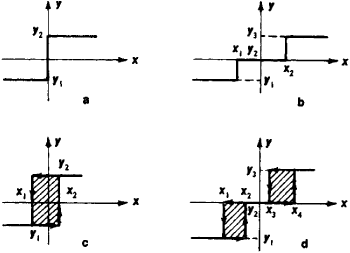# Relay Characteristic

The following article is from The Great Soviet Encyclopedia (1979). It might be outdated or ideologically biased.

## Relay Characteristic

a characteristic curve having a piecewise-linear shape and corresponding to the conversion by a device (or system) of a continuous input variable x into discrete values yn of an output variable y, where η is the number of possible values or levels of the output variable; usually n is equal to 2 or 3. Thus, the curve characterizes the operation of a relay.

Figure 1,a and b shows the characteristics for ideal two-step (n = 2) and three-step (n = 3) relay elements. Figure 1,c and dFigure 1. Relay characteristics: (a) and (c) two-step relay elements, (b) and (d) three-step relay elements

shows the characteristics for actual relay elements. Such characteristics of actual relays have a hysteresis loop (zone of ambiguity): when x varies within the region x, ≤ x < x2 (Figure 1,c) or the regions x1xx2 and x3xx4 (Figure 1,d), the behavior of the function y(x) depends not only on the value of x but on the direction of the change in x. A value of x for which y jumps from one value to another is called an operation threshold. Figure 1,c illustrates the characteristic of, for example, simple two-step electromagnetic relays. The characteristic in Figure 1,d is exhibited by three-step polarized relays. Devices that have relay characteristics are widely used in amplitude quantizers and in relay automatic control systems.

### REFERENCES

See references under relay element.

A. V. KOCHEROV

The Great Soviet Encyclopedia, 3rd Edition (1970-1979). © 2010 The Gale Group, Inc. All rights reserved.
Mentioned in ?
References in periodicals archive ?
and this shows the dependency of distance relay characteristic to load angle, as shown in above equations.onnected to bus A.
It can be seen that, when short circuit level changes, the characteristic will be weakly constant, and this shows the dependency of distance relay characteristic to short circuit level, as shown in above equations.
In this paper, the coordination optimization problem is represented by a nonlinear relay characteristic function and a range of nonlinear coordination constraints.
Relay Characteristics. The nonlinear relay characteristics function based on standard IEEE C37.112-1996 can be stated as follows:
But after you look at the parts, you must combine and analyze overall relay characteristics so you can properly include one of these devices in a circuit as a whole.

Site: Follow: Share:
Open / Close